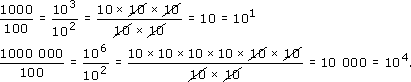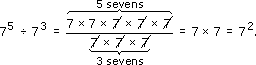Science, Maths & Technology

### Become an OU studentSquares, roots and powers

Start this free course now. Just create an account and sign in. Enrol and complete the course for a free statement of participation or digital badge if available.

# 2.4 Dividing powers

Now consider division in the same way. For example:So 103 ÷ 102 = 101 and 106 ÷ 102 = 104, which suggests that dividing the powers of ten means subtracting the powers. You can see that the two tens underneath have cancelled with two of the tens on top in each case, which shows why the number of tens underneath is subtracted from the number on top. This argument applies equally well to any number. For example:Work out 75 ÷ 73 on your calculator to check this.

To divide two powers of the same number, subtract the powers.